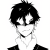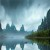# PHP并发下安全读写文件函数

mengkun 18.6K

<?php

/**
* @author warlee | e-mail:kodcloud@qq.com
*/

/**
* 安全读取文件，避免并发下读取数据为空
*
* @param $file 要读取的文件路径 * @param$timeout 读取超时时间
* @return 读取到的文件内容 | false - 读取失败
*/
function file_read_safe($file,$timeout = 5) {
if (!$file || !file_exists($file)) return false;
$fp = @fopen($file, 'r');
if (!$fp) return false;$startTime = microtime(true);

// 在指定时间内完成对文件的独占锁定
do {
$locked = flock($fp, LOCK_EX | LOCK_NB);
if (!$locked) { usleep(mt_rand(1, 50) * 1000); // 随机等待1~50ms再试 } } while ((!$locked) && ((microtime(true) - $startTime) <$timeout));

if ($locked && filesize($file) >= 0) {
$result = @fread($fp, filesize($file)); flock($fp, LOCK_UN);
fclose($fp); if (filesize($file) == 0) {
return '';
}
return $result; } else { flock($fp, LOCK_UN);
fclose($fp); return false; } } /** * 安全写文件，避免并发下写入数据为空 * * @param$file 要写入的文件路径
* @param $buffer 要写入的文件二进制流（文件内容） * @param$timeout 写入超时时间
* @return 写入的字符数 | false - 写入失败
*/
function file_write_safe($file,$buffer, $timeout = 5) { clearstatcache(); if (strlen($file) == 0 || !$file) return false; // 文件不存在则创建 if (!file_exists($file)) {
@file_put_contents($file, ''); } if(!is_writeable($file)) return false;    // 不可写

// 在指定时间内完成对文件的独占锁定
$fp = fopen($file, 'r+');
$startTime = microtime(true); do {$locked = flock($fp, LOCK_EX); if (!$locked) {
usleep(mt_rand(1, 50) * 1000);   // 随机等待1~50ms再试
}
}
while ((!$locked) && ((microtime(true) -$startTime) < $timeout)); if ($locked) {
$tempFile =$file.'.temp';
$result = file_put_contents($tempFile, $buffer, LOCK_EX); if (!$result || !file_exists($tempFile)) { flock($fp, LOCK_UN);
fclose($fp); return false; } @unlink($tempFile);

ftruncate($fp, 0); rewind($fp);
$result = fwrite($fp, $buffer); flock($fp, LOCK_UN);
fclose($fp); clearstatcache(); return$result;
} else {
flock($fp, LOCK_UN); fclose($fp);
return false;
}
}

## 后记

1.小星云 Lv 3

大佬，并发下写文件会丢失数据，只读文件也会读不到吗[疑问]

•mengkun 站长

@小星云只读取不会

•小星云 Lv 3

@mengkun哦[憨笑]谢谢大佬，我看到这段代码的注释“安全读取文件，避免并发下读取数据为空”还以为会读不到呢！